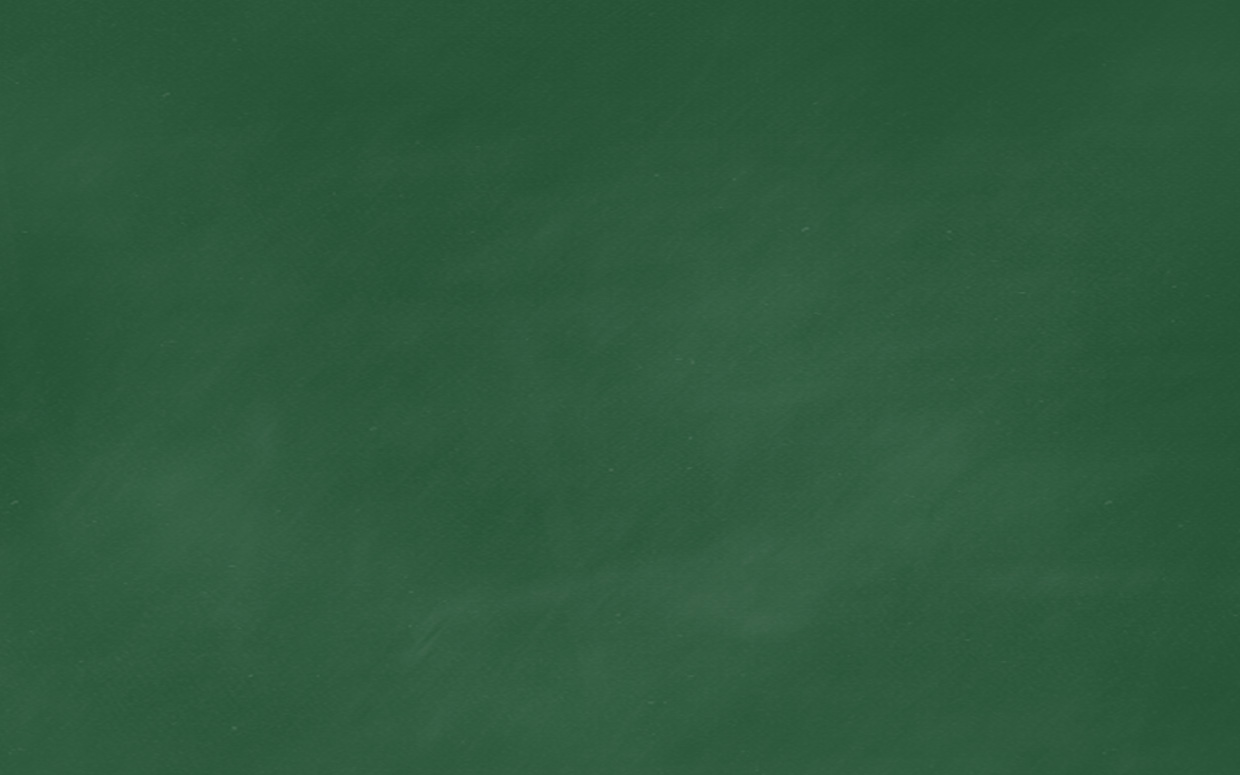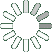Puzzle Command
Daily Puzzle
Number Logic #3
December 6, 2014Find a 4 digit number where:

• The 1st digit is the sum of the 2nd, 3rd, and 4th number.
• The 2nd digit is the number of 2s in the number.
• The 3rd digit is equal to 4.
• The 4th digit is the sum of the 2nd and 3rd number.
Puzzle Notes
4 digit numbers range from 1,000 to 9,999.
Some number logic puzzles have more than one correct answer.
This number logic puzzle only has one correct answer.
Enter in your solution below and click submit.